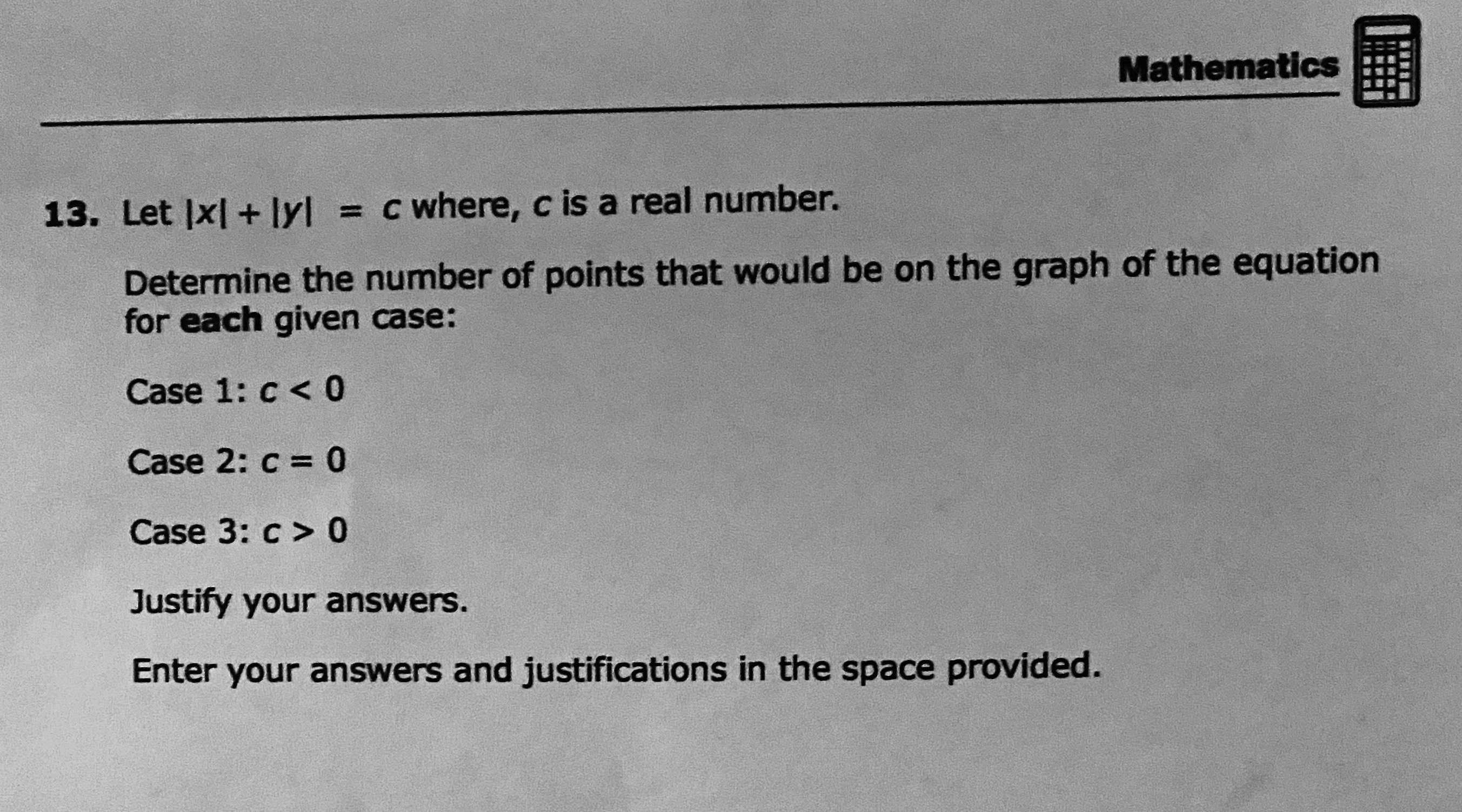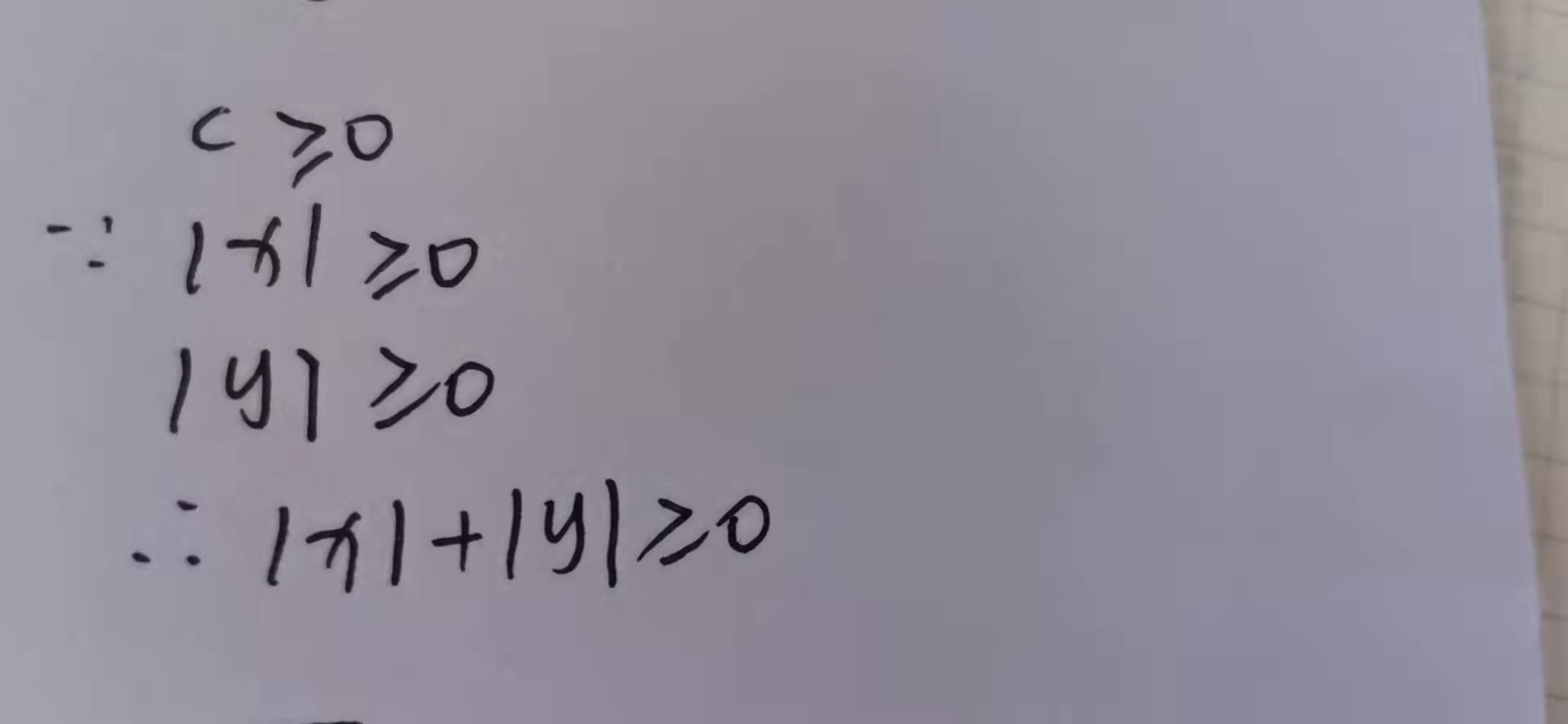### ¿Todavía tienes preguntas de matemáticas?

Pregunte a nuestros tutores expertos
Algebra
Pregunta13. Let $$| x | + | y | = c$$ where, $$c$$ is a real number. Determine the number of points that would be on the graph of the equation for each given case:

Case $$1 : c < 0$$

Case $$2 : c = 0$$

Case $$3 : c > 0$$